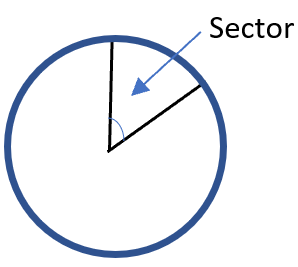# Math Worksheets Land

Math Worksheets For All Ages

# Math Worksheets Land

Math Worksheets For All Ages

Home > Grade Levels > High School Geometry >

# Area of a Sector Worksheets

We have already learned how to determine the area of a circle. To calculate it for any circle we just determine the radius and plug it into the formula for the area of circle (πr2). What if we were only interested in a slice of that circle such as the shape of your favorite tasty pie or pizza? We call these slices sectors. A sector is defined as a portion of the circumference of the circle and 2 radii of the circle meet at both endpoints of the arc. When you consider any sector, it breaks the circle into two sectors; the slice (smaller section) is referred to as the minor sector and remainder of the circle is referred to as the major sector. Depending on how the units of measure used for angle theta determines the formula used to calculate the area of a sector. Students learn how to determine this measure of sector of a circle with these worksheets and lessons.

### Aligned Standard: High School Geometry - HSG-C.B.5

• Answer Keys - These are for all the unlocked materials above.

### Homework Sheets

I have always found this topic fascinating. I always wondered how they calculated linear feet in round structures.

• Homework 1 - We work on determining the measures where K is the area of the sector, A is the area of the circle, and m is the measure in degrees of the arc bounding the sector.
• Homework 2 - The formula for the area of a sector is: K = m / 360 degrees.
• Homework 3 - We breakdown the formula into components and work it through ending by multiplying and simplifying.

### Practice Worksheets

I learned from my neighbor that they teach this skill to snipers in the Marines. I guess I can see the relevance.

• Practice 1 - The radius of circle is 8 miles. What is the area of a sector bounded by a 10 degree arc?
• Practice 2 - These are much larger slices of pie here. They cover large distances.
• Practice 3 - Find the area of sector right away. We ramp up the measures of the angles here.

### Math Skill Quizzes

It's all about the area of parts of circles.

• Quiz 1 - Use the blue outline in grayscale mode. It will help make it pop for you.
• Quiz 2 - This is really helpful to figure out who eats the most pizza.
• Quiz 3 - These pies are full of great stuff. The angles we work with here are very large.

### How Do You Find the Area of a Sector of a Circle?Do you love pizza? Have you ever wondered what the shape of a slice of pizza is called? No, it is not a triangle but a sector of a circle. A sector of a circle is the region that is bounded by two radii and an arc.

Finding the area of the sector is easy and can be calculated using a simple formula.

There are two formulas that can be used to calculate this measure of the sector.

This is the area of circle times the fraction of the circle the sector represents.

Area = n /360 × πr2

Here n is the angle of that exact region. The formula is used when the arc length is not given.

When the arc length is given, you can use the following formula to calculate the area of a sector.

Area = s / 2πr × πr2. A sector of a circle is just like a slice of pizza or pie.

### Where Do You Use This in The Real World?There are hundreds of measures of circles that are imperative to many industries. Being able to pinpoint the area of sector of that circle is a paramount skill for these industries. I know on these worksheets we are focused on the two-dimensional aspects of circles, but if we were to ponder these objects in three dimensions, you will quickly see the application to the industrial world. Think of something that is a circle. How about car tires? Car tires are three dimensional circles that are made of rubber, fabric, wire, along with a series of proprietary chemical compounds. If you were to manufacture your own tires it would be critical to know how much area you would need to cover to mold each tire. That measure would determine how many raw materials you would need. Know that, it would allow you to estimate the cost of the tire and give you idea of how much to charge your customers. Have you ever seen a well-maintained field that has elaborate sprinkler systems? The architects that design those systems need to understand the area that surrounds each sprinkler, which area needs to be watered, and the section of the three hundred and sixty degree of radius that it is responsible for.

Unlock all the answers, worksheets, homework, tests and more!
Save Tons of Time! Make My Life Easier Now

## Thanks and Don't Forget To Tell Your Friends!

I would appreciate everyone letting me know if you find any errors. I'm getting a little older these days and my eyes are going. Please contact me, to let me know. I'll fix it ASAP.